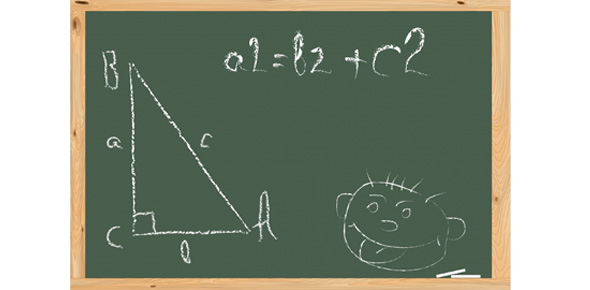# Square Roots And Pythagorean Theorem

9 Questions | Attempts: 471
ShareSettingsComplete the following expressions and equations involving square roots and pythagorean theorem.

• 1.
What are the two square roots of 64?
• A.

32, -32

• B.

16, -16

• C.

8, -8

• 2.
√  81
• A.

9

• B.

-9

• C.

Both 9 and -9

• 3.
Which integer is a perfect square?
• A.

1

• B.

2

• C.

3

• 4.
Approximate the square root of 10
• A.

Beteen 4 and 5

• B.

Berween 3 and 4

• C.

Berween 2 and 3

• 5.
- √256
• A.

16

• B.

-16

• C.

Both 16 and -16

• 6.
Approximate the square root of 161
• A.

Between 12 and 13

• B.

Bewteen 13 and 14

• C.

Between 14 and 15

• 7.
Find the hypotenuse of a right triangle whose legs are 5 and 6.
• A.

6.5

• B.

7

• C.

7.8

• 8.
Find the hypotenuse of a right triangle whose legs are 30 and 40
• A.

50

• B.

55

• C.

125

• 9.
Check all the boxes that list possible side lengths for right triangles
• A.

12, 15, 16

• B.

3, 4, 7

• C.

18, 22, 30

## Related TopicsBack to top
×

Wait!
Here's an interesting quiz for you.